# Image Segmentation By Clustering

• Last Updated : 18 Jul, 2021

Segmentation By clustering

It is a method to perform Image Segmentation of pixel-wise segmentation. In this type of segmentation, we try to cluster the pixels that are together. There are two approaches for performing the Segmentation by clustering.

Attention reader! Don’t stop learning now. Get hold of all the important Machine Learning Concepts with the Machine Learning Foundation Course at a student-friendly price and become industry ready.

• Clustering by Merging
• Clustering by Divisive

Clustering by merging or Agglomerative Clustering:

In this approach, we follow the bottom-up approach, which means we assign the pixel closest to the cluster. The algorithm for performing the agglomerative clustering as follows:

• Take each point as a separate cluster.
• For a given number of epochs or until clustering is satisfactory.
• Merge two clusters with the smallest inter-cluster distance (WCSS).
• Repeat the above step

The agglomerative clustering is represented by Dendrogram. It can be performed in 3 methods: by selecting the closest pair for merging, by selecting the farthest pair for merging, or by selecting the pair which is at an average distance (neither closest nor farthest). The dendrogram representing these types of clustering is below: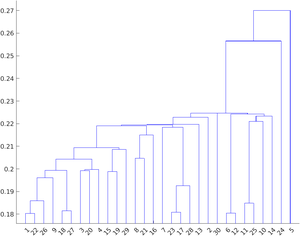Nearest clustering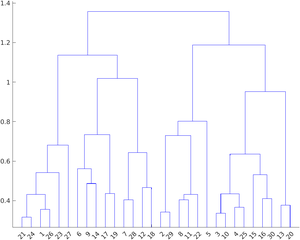Average Clustering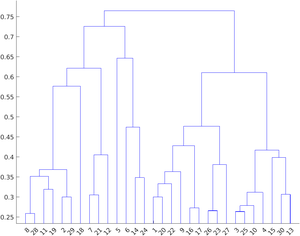Farthest Clustering

Clustering by division or Divisive splitting

In this approach, we follow the top-down approach, which means we assign the pixel closest to the cluster. The algorithm for performing the agglomerative clustering as follows:

• Construct a single cluster containing all points.
• For a given number of epochs or until clustering is satisfactory.
• Split the cluster into two clusters with the largest inter-cluster distance.
• Repeat the above steps.

In this article, we will be discussing how to perform the K-Means Clustering.

K-Means Clustering

K-means clustering is a very popular clustering algorithm which applied when we have a dataset with labels unknown. The goal is to find certain groups based on some kind of similarity in the data with the number of groups represented by K. This algorithm is generally used in areas like market segmentation, customer segmentation, etc. But, it can also be used to segment different objects in the images on the basis of the pixel values.

The algorithm for image segmentation works as follows:

1. First, we need to select the value of K in K-means clustering.
2. Select a feature vector for every pixel (color values such as RGB value, texture etc.).
3. Define a similarity measure b/w feature vectors such as Euclidean distance to measure the similarity b/w any two points/pixel.
4. Apply K-means algorithm to the cluster centers
5. Apply connected component’s algorithm.
6. Combine any component of size less than the threshold to an adjacent component that is similar to it until you can’t combine more.

Following are the steps for applying the K-means clustering algorithm:

• Select K points and assign them one cluster center each.
• Until the cluster center won’t change, perform the following steps:
• Allocate each point to the nearest cluster center and ensure that each cluster center has one point.
• Replace the cluster center with the mean of the points assigned to it.
• End

#### The optimal value of K?

For a certain class of clustering algorithms, there is a parameter commonly referred to as K that specifies the number of clusters to detect. We may have the predefined value of K, if we have domain knowledge about data that how many categories it contains. But, before calculating the optimal value of K, we first need to define the objective function for the above algorithm. The objective function can be given by:Where j is the number of clusters, and i will be the points belong to the jth cluster. The above objective function is called within-cluster sum of square (WCSS) distance.

A good way to find the optimal value of K is to brute force a smaller range of values (1-10) and plot the graph of WCSS distance vs K. The point where the graph is sharply bent downward can be considered the optimal value of K. This method is called Elbow method.

For image segmentation, we plot the histogram of the image and try to find peaks, valleys in it. Then, we will perform the peakiness test on that histogram.

### Implementation

• In this implementation, we will be performing Image Segmentation using K-Means clustering. We will be using OpenCV k-Means API to perform this clustering.

## Python3

 # importsimport numpy as npimport cv2 as cvimport matplotlib.pyplot as plt  plt.rcParams["figure.figsize"] = (12,50)  # load imageimg = cv.imread('road.jpg')Z = img.reshape((-1,3))# convert to np.float32Z = np.float32(Z)  # define stopping criteria, number of clusters(K) and apply kmeans()# TERM_CRITERIA_EPS : stop when the epsilon value is reached# TERM_CRITERIA_MAX_ITER:  stop when Max iteration is reachedcriteria = (cv.TERM_CRITERIA_EPS + cv.TERM_CRITERIA_MAX_ITER, 10, 1.0)  fig, ax = plt.subplots(10,2, sharey=True)for i in range(10):  K = i+3  # apply K-means algorithm  ret,label,center=cv.kmeans(Z,K,None,criteria,attempts = 10,                             cv.KMEANS_RANDOM_CENTERS)  # Now convert back into uint8, and make original image  center = np.uint8(center)  res = center[label.flatten()]  res2 = res.reshape((img.shape))  # plot the original image and K-means image  ax[i, 1].imshow(res2)  ax[i,1].set_title('K = %s Image'%K)  ax[i, 0].imshow(img)  ax[i,0].set_title('Original Image')

Output: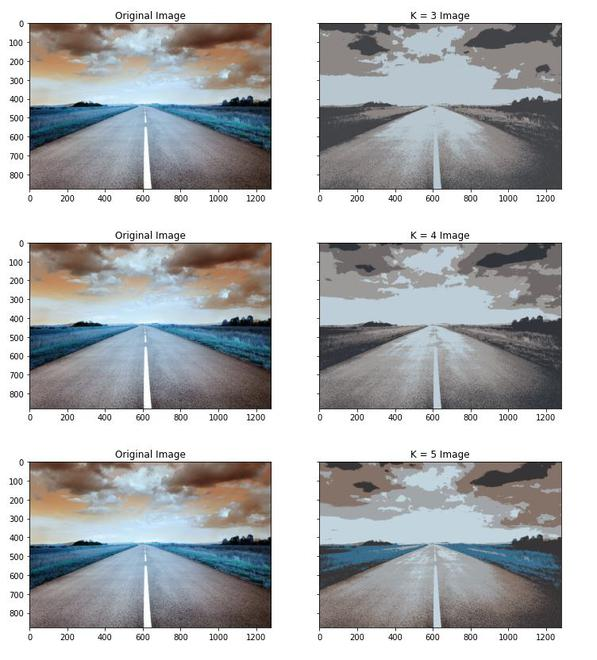Image Segmentation for K=3,4,5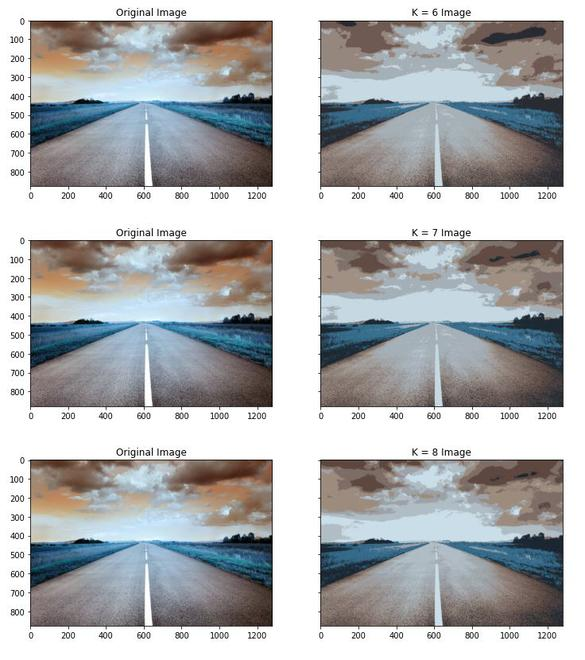Image Segmentation for K=6,7,8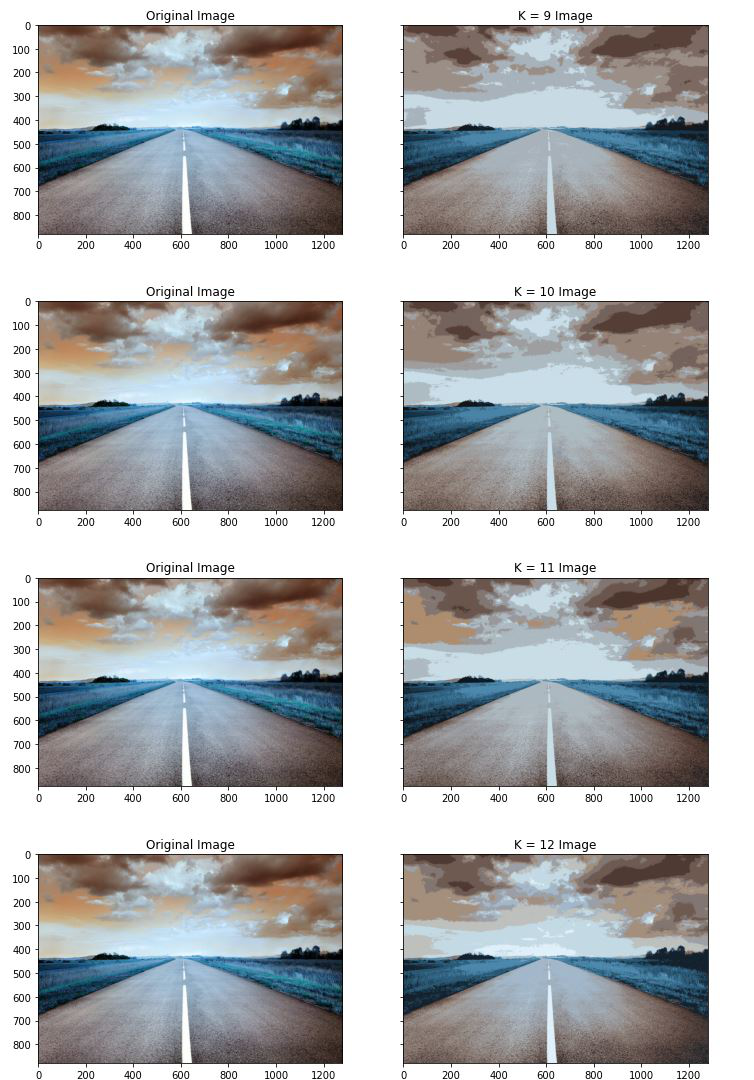### References:

My Personal Notes arrow_drop_up